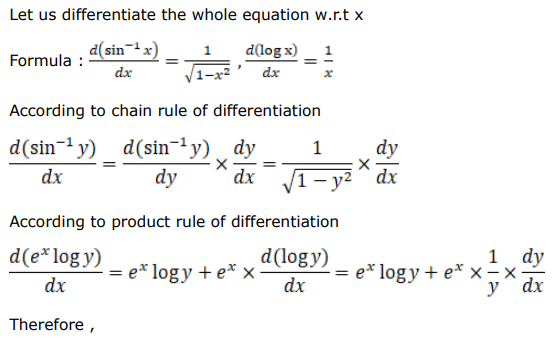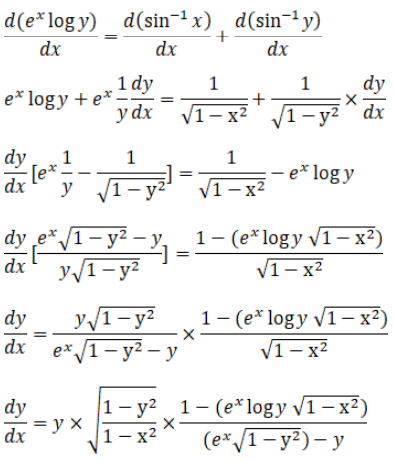# Solve this followingQuestion:

Find, when:

$e^{x} \log y=\sin ^{-1} x+\sin ^{-1} y$

Solution: